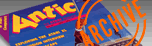`ANTIC VOL. 4, NO. 4 / AUGUST 1985`

# EXTRA

(Several short BASIC programs included on the back of the magazine subscription card page as a bonus)

More colorful enjoyment for your Atari from Antic. Each of these BASIC programs is a mini computer artwork, just for you!

``````
1 PRINT "[ESC][SHIFT][CLEAR]WHAT GRAPHICS MODE(9,10,11)";:INPUT A
29 REM LIGHTSHOW #2
30 GRAPHICS A:FOR I=0 TO 79:FOR J=0 TO 79:COLOR I+J/4+4
40 PLOT I,79-J:PLOT J,79-I:PLOT 79-J,79-I:PLOT 79-I,79-J:PLOT 79-I,J:PLOT 9-J,I:PLOT I,J:PLOT J,I
50 NEXT J:NEXT I
60 GOTO 60

5 Z=0
10 GRAPHICS 11
20 FOR I=1 TO 79
30 Z=Z+1:IF Z>9 THEN Z=1
35 COLOR Z
40 PLOT I,X:DRAWTO 79-I,X
50 PLOT I,190-X:DRAWTO 79-I,190-X
60 X=X+2:IF X>190 THEN X=0
70 NEXT I:Z=Z+1:GOTO 10

10 GRAPHICS 8+16
20 SETCOLOR 2,10,0
30 FOR I=1 TO 190 STEP 7
40 PLOT I,0:DRAWTO 300,I
45 PLOT 300-I,190:DRAWTO 0,190-I
50 PLOT 0,190-I:DRAWTO I,0
55 PLOT 300,I:DRAWTO 300-I,190
60 NEXT I
65 GOTO 65

``````10 GRAPHICS 11
20 FOR X=0 TO 70
30 C=C+1:COLOR C:IF C=127 THEN C=0
40 PLOT X,Y:DRAWTO 78-X,Y:DRAWTO 78-X,191-Y:DRAWTO X,191-Y:DRAWTO X,Y
50 Y=Y+2:IF Y>191 THEN Y=0
60 NEXT X:GOTO 20
``````